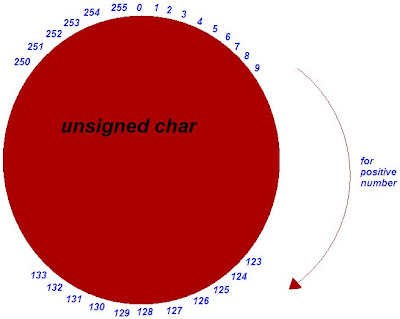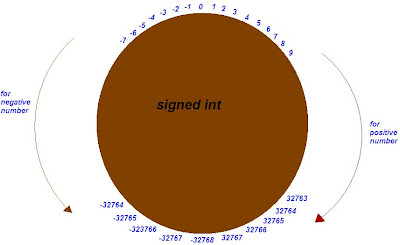## INDEX

### Cyclic nature of data type in C

In C some data types shows one special properties that when we assign a value beyond range of that data type then it will not any compiler error but assign a number according to some cyclic order. This property is known as cyclic nature of data type.
Data type which shows cyclic nature:
(a) char
(b) int
(c) long int
Data type which doesn’t show cyclic nature:
(a) float
(b) double
(c) long double

1. Cyclic nature of unsigned char:
Range of unsigned char is 0 to 255. If we will assign a value greater than 255 then value of variable will be changed to a value if we will move clockwise direction as shown in the figure according to number. If number is less than 0 then move in anti clockwise direction.Short cut formula to find cyclic value:
If number is X where X is greater than 255 then
New value = X % 256
If number is Y where Y is less than 0 then
New value = 256 – (Y% 256)

2. Cyclic nature of signed
char:

Range of unsigned char is -128 to 127. If we will assign a value greater than 127 then value of variable will be changed to a value if we will move clockwise direction as shown in the figure according to number. If number is less than -128 then move in anti clockwise direction.

Short cut formula to find cyclic value:
If number is X where X is greater than 127 then
p = X % 256
if p <=127
New value = p
else
New value = p – 256
If number is Y where Y is less than -128 then
p = Y % 256
If p <= 127
New value = -p
else
New value = 256 -p

3. Cyclic nature of unsigned int:

Range of unsigned int is 0 to 65535. If we will assign a value greater than 65535 then value of variable will be changed to a value if we will move clockwise direction as shown in the figure according to number. If number is less than 0 then move in anti clockwise direction.Short cut formula to find cyclic value:
If number is X where X is greater than 65535 then
New value = X % 65536
If number is Y where Y is less than 0 then
New value = 65536– (Y% 65536)

4. Cyclic nature of signed int:

Range of unsigned int is -32768 to 32767. If we will assign a value greater than 32767 then value of variable will be changed to a value if we will move clockwise direction as shown in the figure according to number. If number is less than -32768 then move in anti clockwise direction.If number is X where X is greater than 32767 then
p = X % 65536
if p <=32767

New value = p
else
New value = p - 65536
If number is Y where Y is less than -32768 then
p = Y % 65536
If p <= 32767
New value = -p
else
New value = 65536 -p

Note: Same rule is also applicable in case of signed long int and unsigned long int.Anonymous said...

#include
#include
void main()
{
char a=200;
pintf("%d",a);
getch();
}
o/p is -56...how????plzz explain.....

Inwhich category he comes 1,2,3

Kk said...

subtract 127 from 200 and add result to -128... u vl get -56 as ans coz of cyclic property.
u didn't specify whether a is signed or unsigned so by default compiler is taking it as signed...kiru said...

Really very good and easy to understand

Unknown said...

hey,it was very helpful.but you wrote 653535 instead of 65535 in point 3 and ubsigned instead of signed in point 4.:)

Unknown said...

Thanks a ton for posting good questions that help widen our understanding of how computers work

Unknown said...

Awesome explanation.....a ton of thanks to u .....it greatly helped me understand C

Unknown said...

Int main()
{
Char str1[]="pratik";
Char str2[]="pratik";
If(str1==str2)
print("equal\n");
else
printf("unequal\n");
return 0;
}
What will be the output??

Unknown said...

Int main()
{
Char str1[]="pratik";
Char str2[]="pratik";
If(str1==str2)
print("equal\n");
else
printf("unequal\n");
return 0;
}
What will be the output??